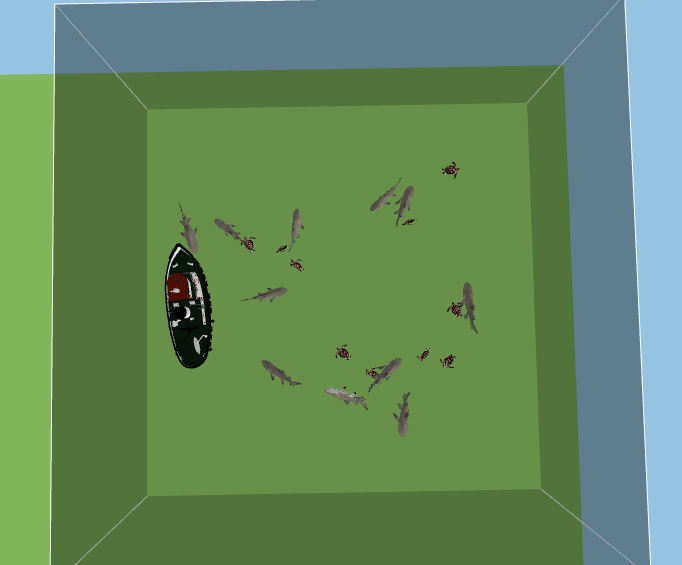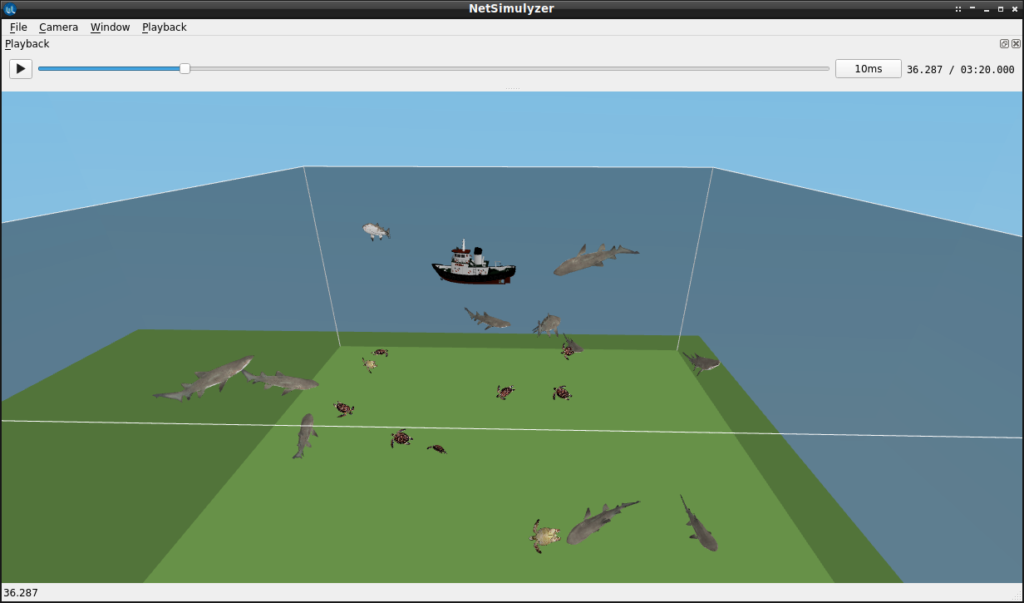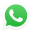# Implementation of Circle Mobility Model for ns-3 and Visualizing it in 3D

## Mobility Models of ns-3.The default ns-3 installation will contain the following mobility models.

#### MobilityModel Subclasses

• ConstantPosition
• ConstantVelocity
• ConstantAcceleration
• GaussMarkov
• Hierarchical
• RandomDirection2D
• RandomWalk2D
• RandomWaypoint
• Waypoint

#### PositionAllocator

Position allocators are used at the beginning, to set the layout of the nodes’ initial position. However, some mobility models will use a position allocator to pick new waypoints.

• ListPositionAllocator
• GridPositionAllocator
• RandomRectanglePositionAllocator
• RandomBoxPositionAllocator
• RandomDiscPositionAllocator
• UniformDiscPositionAllocator

But, we can not simulate circular movements using any of the above models. But sometimes, a simulation may need a node to move in a perfectly circular path.  The 3D output demonstrates two types of mobility models. The Sharks and Turtles are moving in the water using the Gauss-MorkovMobilityModel which is readily available in ns-3. But the Boat which is roaming on the water in a circular path is simulated using the Proposed, Simple CircleMobilityModel.

In this tutorial, I show a new mobility model class implementation for simulating circular mobility under ns-3. Even though a 3D visualization or even 2D visualization is not at all needed while doing scholarly research work, while learning the fundamentals of a simulation, for better understanding, all of us just want to visualize the network that we are simulating. For that, we need a better visualization of the simulated network. In addition to that, the pictorial and video outputs of the simulated network will be useful to make one understand our ideas during our project or research presentations.

### Caution:

This simulation only addresses the primitive problems in simulating and visualizing 3D UWSN Simulation and Mobility models. There are other aspects such as setting different routing protocols and different traffic types in such a UWSN scenario. They are not addressed in this article. Further, there are some additional aspects needed to do a trace analysis on the simulated UWSN scenario; that is also not discussed here. Further, for simulating realistic movements and orientation of 3d nodes, we have to do some modifications in the core Netsimulyzer. That is also not described here.

## The Main Idea of the Design of a  New Mobility Model.

We can easily implement a new, simple mobility model by closing one of the existing mobility models and including the desired functionality in it.  For our design of “CircleMobilityModel”, I simply cloned one of the simplest mobility models called “ConstantPositionMobilityModel” and include the circular mobility functionality in it.

## The “CircleMobilityModel” Class of our “circle-mobility-model.h” file

The following are the important member functions and variables that are declared in CircleMobilityModel class of the header files circle-mobility-model.h.

void SetParameters(const Vector &Origin, const double Radius, const double StartAngle, const double Speed);
void Test(void);

virtual Vector DoGetPosition (void) const;
virtual void DoSetPosition (const Vector &position);
virtual Vector DoGetVelocity (void) const;

Vector m_position; //!< the constant position
Vector m_Origin;
double m_StartAngle;
double m_Speed;
Time m_lastUpdate

## The Important functions of our “circle-mobility-model.cc” file

The following function SetParameters will be called from the simulation script to set the required parameters of the circular mobility.

The parameters to create circle mobility are:

1. Origin of the Circle
2. The radius of the Circle
3. Starting Angle,
4. Speed

void
CircleMobilityModel::SetParameters(const Vector &Origin, const double Radius, const double StartAngle, const double Speed)
{
DoSetPosition(Origin);
m_Origin=Origin;
m_StartAngle=StartAngle;
m_Speed=Speed;
NotifyCourseChange ();
}

## The Function DoGetPosition

The following function will be called in regular intervals by the parent class of the mobility model and make the nodes move to position accordingly in an ns-3 simulation.

Sorry!!!. Some sections are hidden; because, you are only having restricted access to this article.

While getting paid support/assistance for your research from Charles, you may get an access key/password to some articles. If you are having the access key/password to this particular article, then please enter it below to unlock the restricted section of this article.

If your research is related to this area, and you want to get paid support/assistance from Charles, then you may start a 'free' research discussion with Charles.

The following function will call NotifyCourseChange while setting the position of the node.

void
CircleMobilityModel::DoSetPosition (const Vector &position)
{
m_position = position;
m_lastUpdate = Simulator::Now ();
NotifyCourseChange ();
}

The following function will be called in regular intervals by the parent class of the mobility model so that we can get the velocity of the nodes in an ns-3 simulation.

Vector
CircleMobilityModel::DoGetVelocity (void) const
{

Time now = Simulator::Now ();
NS_ASSERT (m_lastUpdate <= now);
//m_lastUpdate = now;
double angle = m_StartAngle+ ((m_Speed/m_Radius) * now.GetSeconds());
double cosAngle = cos(angle);
double sinAngle = sin(angle);

return Vector ( -sinAngle * m_Speed, cosAngle * m_Speed, 0.0);
}

## The Components of the Simple 3D UWSN ns-3 Simulation Script

The following code segment presents the important components of the ns-3 simulation script which is used to simulate and test our new circle mobility model. In this simulation, except the sink node (Boat at the water surface)  all other nodes will use the Gauss-Markov Mobility model. The Boat that is at the water surface will move in a perfect circle using the new “CircleMobilityModel”.

### Include the header files Needed

The following lines include the necessary header files. The suitable 3D mobility model should be incorporated in mobility-module.h and the Netsimulyzer ns-3 module should be installed in the ns-3 directory tree. The header file for aqua-sim-ng and other header files for required components are included.

#include “ns3/core-module.h”
#include “ns3/network-module.h”
#include “ns3/aqua-sim-ng-module.h”
#include “ns3/applications-module.h”
#include “ns3/log.h”
#include “ns3/callback.h”
#include “ns3/mobility-module.h”
#include “ns3/netanim-module.h”
#include “ns3/netsimulyzer-module.h”

The following lines show some of the parameters that we used for creating different nodes and setting their communication attributes for this underwater network simulation.

double simStop = 20; //seconds
uint32_t noShark = 10;
uint32_t noTurtles = 10;
uint32_t m_dataRate = 10000;
uint32_t m_packetSize = 40;
double range = 100;

NodeContainer SharkCon;
NodeContainer TurtlesCon;
NodeContainer sinksCon;
NodeContainer senderCon;
SharkCon.Create(noShark);
TurtlesCon.Create(noTurtles);
sinksCon.Create(1);
senderCon.Create(1);

PacketSocketHelper socketHelper;
socketHelper.Install(SharkCon);
socketHelper.Install(TurtlesCon);
socketHelper.Install(sinksCon);
socketHelper.Install(senderCon);

### Simulate some 3D Ocean Water Body top of the Ocean Ground

The following section of code will mimic some water body for our UWSN. (This will not affect the Physical Media Implementation of AquaSim NG – it is just for 3D visualization on NetSimulyzer only.

// create a some Water Body
double WaterSizeX = 500; // m
double WaterSizeY = 500; // m
double WaterHeight = 250; // m

std::vector<Ptr<Building> > WaterVector;

Ptr < Building > Water;
Water = CreateObject<Building> ();

Water->SetBoundaries (Box (0,
WaterSizeX,
0,
WaterSizeY,
0.0, WaterHeight));
Water->SetNRoomsX (1);
Water->SetNRoomsY (1);
Water->SetNFloors (1);
WaterVector.push_back (Water);

### Create Some Mobile Underwater Sensor Nodes that were attached on Sharks and Turtles and install Gauss Markov 3D Mobility Model them

/*
* Preset up mobility model for nodes and sinks here
*/
MobilityHelper mobility;

mobility.SetMobilityModel (“ns3::GaussMarkovMobilityModel”,
“Bounds”, BoxValue (Box (0, 300, 0, 300, 20, 200)),
“TimeStep”, TimeValue (Seconds (0.5)),
“Alpha”, DoubleValue (0.85),
“MeanVelocity”, StringValue (“ns3::UniformRandomVariable[Min=0|Max=10]”),
“MeanDirection”, StringValue (“ns3::UniformRandomVariable[Min=0|Max=6.283185307]”),
“MeanPitch”, StringValue (“ns3::UniformRandomVariable[Min=0.05|Max=0.5]”),
“NormalVelocity”, StringValue (“ns3::NormalRandomVariable[Mean=10.0|Variance=5.0|Bound=10.0]”),
“NormalDirection”, StringValue (“ns3::NormalRandomVariable[Mean=0.0|Variance=0.2|Bound=0.4]”),
“NormalPitch”, StringValue (“ns3::NormalRandomVariable[Mean=0.0|Variance=0.02|Bound=0.04]”));

mobility.Install (SharkCon);
mobility.Install (senderCon);

MobilityHelper mobilityTurtle;

mobilityTurtle.SetMobilityModel (“ns3::GaussMarkovMobilityModel”,
“Bounds”, BoxValue (Box (0, 300, 0, 300, 20, 70)),
“TimeStep”, TimeValue (Seconds (0.5)),
“Alpha”, DoubleValue (0.85),
“MeanVelocity”, StringValue (“ns3::UniformRandomVariable[Min=0|Max=1]”),
“MeanDirection”, StringValue (“ns3::UniformRandomVariable[Min=0|Max=6.283185307]”),
“MeanPitch”, StringValue (“ns3::UniformRandomVariable[Min=0.05|Max=0.5]”),
“NormalVelocity”, StringValue (“ns3::NormalRandomVariable[Mean=1.0|Variance=5.0|Bound=1.0]”),
“NormalDirection”, StringValue (“ns3::NormalRandomVariable[Mean=0.0|Variance=0.2|Bound=0.4]”),
“NormalPitch”, StringValue (“ns3::NormalRandomVariable[Mean=0.0|Variance=0.02|Bound=0.04]”));

mobilityTurtle.SetPositionAllocator (“ns3::RandomBoxPositionAllocator”,
“X”, StringValue (“ns3::UniformRandomVariable[Min=00.0|Max=300.0]”),
“Y”, StringValue (“ns3::UniformRandomVariable[Min=00.0|Max=300.0]”),
“Z”, StringValue (“ns3::UniformRandomVariable[Min=20.0|Max=70.0]”));
mobilityTurtle.Install (TurtlesCon);

### Create a Sink Node and install “CircleMobilityModel” in it

BoatMobility.SetMobilityModel (“ns3::CircleMobilityModel”);
BoatMobility.Install (sinksCon);
sinksCon.Get (0)->GetObject<CircleMobilityModel> ()->SetParameters(Vector (150, 150, 235), 135, 90, 10);

### Configure Things for 3D Netsimulyzer Visualization

The following are the three important things that will be done by this section of code:

• The 3D simulation output will be stored in the file “AquaticAnimalTracking-NS3UWSNCircleMobilityModel3D.json”.
• The underwater nodes will be represented by a 3D Shark models and Turtle models
• The Sink Node will be represented by a 3d Boat object on the Water Surface
• The 3D water body will be created using the previosuly configured information

auto orchestrator = CreateObject<netsimulyzer::Orchestrator>
(“AquaticAnimalTracking-NS3UWSNCircleMobilityModel3D.json”);
// Use helper to define model for visualizing nodes and aggregate to Node object
netsimulyzer::NodeConfigurationHelper TigerWhalenodeHelper{orchestrator};

TigerWhalenodeHelper.Set (“Model”, netsimulyzer::models::SHARK_VALUE);

TigerWhalenodeHelper.Install(SharkCon);
TigerWhalenodeHelper.Install(senderCon);

netsimulyzer::NodeConfigurationHelper TurtlenodeHelper{orchestrator};

TurtlenodeHelper.Set (“Model”, netsimulyzer::models::TURTLE_VALUE);

TurtlenodeHelper.Install(TurtlesCon);

netsimulyzer::NodeConfigurationHelper sinkHelper{orchestrator};

sinkHelper.Set (“Model”, netsimulyzer::models::BOAT_VALUE);

sinkHelper.Install(sinksCon);

// Use helper to configure water bocy and export them
netsimulyzer::BuildingConfigurationHelper WaterHelper{orchestrator};
for (auto &Water : WaterVector)
WaterHelper.Install(Water);

A view of the network from the top surface. (see the bottom surface of the boat which is on the surface of the water).  You can see the simulated tiger sharks and turtles that were roaming under the water.

###Configure standard NetAnim file and end the simulation

For comparison purposes, we create a NetAnim simulation file, which will only be capable of showing 2D of this 3D Mobile UWSN scenario.

AnimationInterface anim (“AquaticAnimalTracking-NS3UWSNCircleMobilityModel3D.xml”);

Simulator::Stop (Seconds (simTimeSec));
Simulator::Run ();
Simulator::Destroy ();

The following NetAnim output shows the movements of CircleMobilityModel in 2D canvas. For better visibility, the size of the boat node is set a little bit higher than the turtles and the sharks.

### The  3D Circle Mobility Model Demo

The following video shows the 3D output of the Mobile UWSN simulation with CircleMobilityModel on NetSimulyzer. Under this 3D visualization, we can clearly understand the circular motion of the Boat Node.

Note: The visualization of Packet Transmission and wave Propagation on Medium is not yet implemented in NetSimulyzer. So we can only see the movement of nodes. But we can add “log events” to understand the events of transmission and reception through some text messages that will be displayed in a separate text window of NetSimulyzer.

In the following articles, we will extend the functionality of our CircleMobilityModel:

## For Assistance in Protocol Implementation, Simulations & Analysis of Industrial as well as Scholarly Research Works, you may Contact Us.Discuss Through WhatsApp

## Send an e-Mail Message.

This site is protected by reCAPTCHA and the Google